Saturday, June 12, 2021
Home > Latest Announcement > NTSE Maths Previous Year 500 Questions Bank With Solutions PDF Download

# NTSE Maths Previous Year 500 Questions Bank With Solutions PDF Download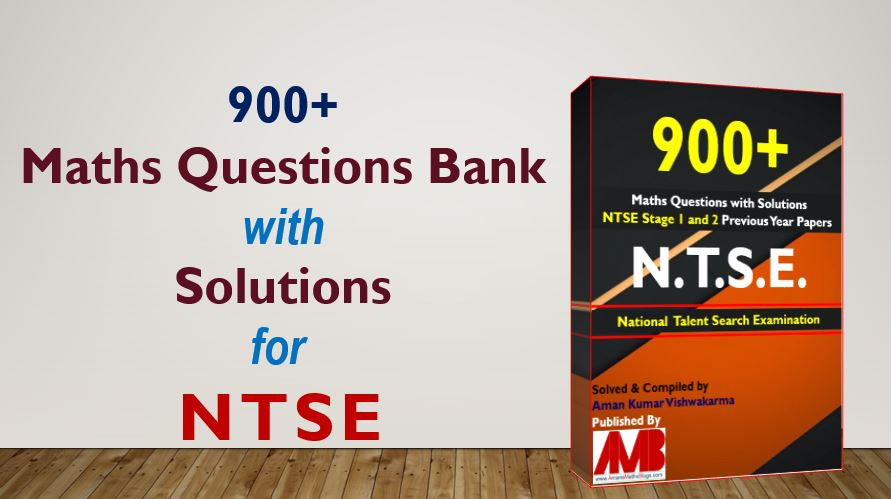# NTSE Maths Previous Year 900 Questions Bank With Solutions

Hi students, welcome to AmansMathsBlogs (AMB). As we know that the NTSE is an acronym for National Talent Search Examination. It is a two stages level exams. NTSE stage 1 is a state level exams and NTSE Stage 2 is a national level exams. NTSE is a national level scholarship program. It is one of the oldest exams in INDIA for CBSE Class 10 students.  NTSE stage 1 and NTSE stage 2, both are conducted by NCERT (National Council of Education Research and Training). So this PDF, NTSE Maths Previous Year 500 Questions Bank With Solutions and Answer Keys is very helpful for NTSE Stage 1 and 2 exams.

[Updated in October 2019)]

As NTSE Stage 1 and Stage 2 exams are conducted by NCERT. It is conducted for identifying the students who are highly intellect and academic talent. The main objective of NTSE scholarship program is recognizing intelligent students and nurturing their talent. So it is one of most prestigious scholarship examination in high school level exams. Thus, this NTSE Maths Previous Year 500 Questions Bank With Solution and Answer Keys PDF is must for the aspiring NTSE students.

Therefore, In this article, you will get the collection of NTSE Maths Previous Year 500 Questions Bank With Solutions and Answer Keys. You can download it as a PDF file. This PDF file contains the maths questions along with answer keys and solutions and all the questions are taken from NTSE previous year exams. These questions are solved by me, AMAN RAJ, I am maths faculty of IIT Maths Foundation course and founder of this website AmansMathsBlogs (AMB).## NTSE Maths Previous Year 900 Questions Bank

NTSE Maths Previous Year 900 Questions Bank With Solutions: Ques No 44

The graphs of the equations x – y = 2 and kx + y = 3, where k is the constant, intersect at the point (x, y) in the first quadrant, if and only if k is

[NTSE Stage-1 2016]

Options:

A. Equal to –1

B. Greater than -1

C. Less than 3/2

D. Lying between –1 and 3/2

Solution:

Downloading Link is At Last

NTSE Maths Previous Year 900 Questions Bank With Solutions: Ques No 113

The perimeter and area of a sector are 18 cm and 20 sq. cm respectively. Then the length of the arc is

[NTSE Stage-1 Tamil Nadu 2014]

Options:

A. 10 cm or 8 cm

B. 10 cm or 5 cm

C. 10 cm or 4 cm

D. 20 cm or 2 cm

Solution:

Downloading Link is At Last

NTSE Maths Previous Year 900 Questions Bank With Solutions: Ques No 132

The length of two parallel chords of a circle are 6 cm and 8 cm. If the smaller chord is at the distance 4 cm from the centre, then the distance of the other chord from the centre is

[NTSE Stage-1 Tamil Nadu 2014]

Options:

A. 5 cm

B. 4 cm

C. 3 cm

D. 2 cm

Solution:

Downloading Link is At Last

NTSE Maths Previous Year 900 Questions Bank With Solutions: Ques No 205

The In ΔABC, AD is median and E is the mid-point of AD. If BE is extended, it meets AC in F. AB = 8 cm, BC = 21 cm and AC = 15 cm, then AF = ?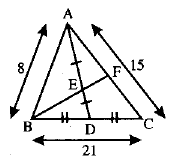[NTSE Stage-1 Jharkhand 2015]

Options:

A. 7 cm

B. 3 cm

C. 12 cm

D. 5 cm

Solution:

Downloading Link is At Last

NTSE Maths Previous Year 900 Questions Bank With Solution: Ques No 233

In the figure below, AB is a diameter of circle and AT is tangent line then the value of x will be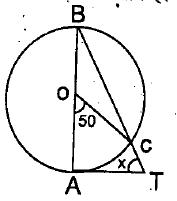[NTSE Stage-1 Chhattisgarh 2014]

Options:

A. 65 degrees

B. 50 degrees

C. 45 degrees

D. 90 degrees

Solution:

Downloading Link is At Last

NTSE Math Previous Year 900 Questions Bank With Solution: Ques No 298

If x and y are two positive real numbers such that their sum is one, then maximum value of x4y + xy4 is

[NTSE Stage-1 Haryana 2014]

Options:

A. 1/6

B. 1/8

C. 1/12

D. 1/16

Solution:

Downloading Link is At Last

NTSE Maths Previous Year 900 Questions Bank With Solution: Ques No 310

ΔABC has vertices A(–4, 1), B(2, –1) and C(1, k). The number of possible values for k such that the triangle is isosceles is

[NTSE Stage-1 Haryana 2014]

Options:

A. 1

B. 3

C. 5

D. 4

Solution:

Downloading Link is At Last

NTSE Maths Previous Year 900 Questions Bank With Solution: Ques No 364

A person walked diagonally across a square plot. Approximately, what was the percentage saved by not walking along the edges?

[NTSE Stage-1 Karnataka 2014]

Options:

A. 35%

B. 30%

C. 20%

D. 25%

Solution:

Downloading Link is At Last

NTSE Maths Previous Year 900 Questions Bank With Solution: Ques No 400

As shown in the figure on the right angled triangle ABC is divided into six smaller triangles by lines drawn from the vertices through a common interior point. The areas of four of these triangles are indicated in the figure. Then, the area of the triangle is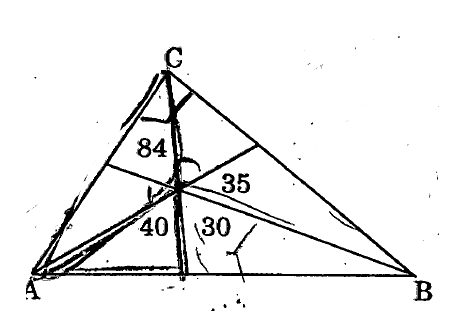[NTSE Stage-1 Andhra Pradesh 2014]

Options:

A. 315

B. 240

C. 275

D. 185

Solution:

Downloading Link is At Last

NTSE Maths Previous Year 900 Questions Bank With Solution: Ques No 469

Let A, M and C be non-negative integers such that A + M + C = 12. What is the maximum value of AMC + AM + MC + CA?

[NTSE Stage-1 Telangana 2013]

Options:

A. 112

B. 62

C. 72

D. 92

Solution:

NTSE Maths Previous Year 900+ Questions Bank With Solution PDF

(Click Here TO Get This PDF)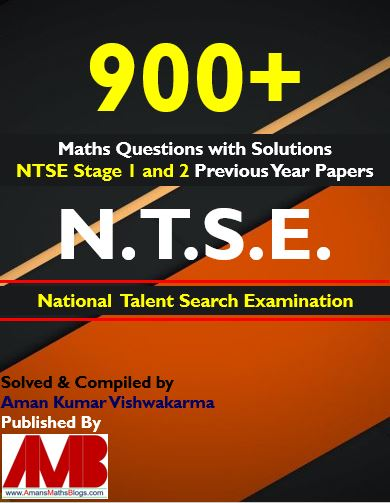[In updated PDF EBook: No of Pages = 877 and File Size = 52MB]

Get File: Free PDF of 129 Maths Short Tricks (Click On Image)## NTSE Maths Combo Pack EBook

Get NTSE Maths Previous Year Question Bank with 900+ Questions with Solutions and 11 Mock Test Papers EBook for NTSE exams. For more details, Click To NTSE Stage 1 and NTSE Stage 2. To get this combo ebook, click below RED button.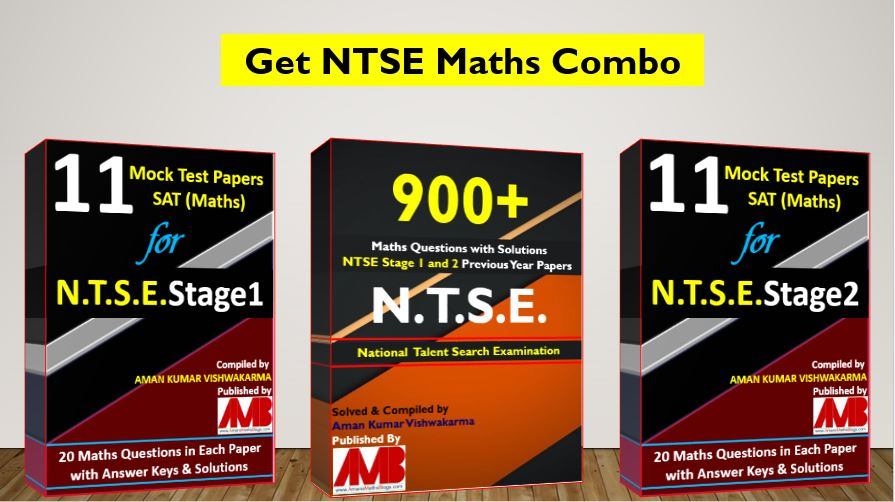900+ Math Questions Bank & 11 Mock Test Paper for NTSE Stage 1 & 2 SAT (Maths)

error: Content is protected !!